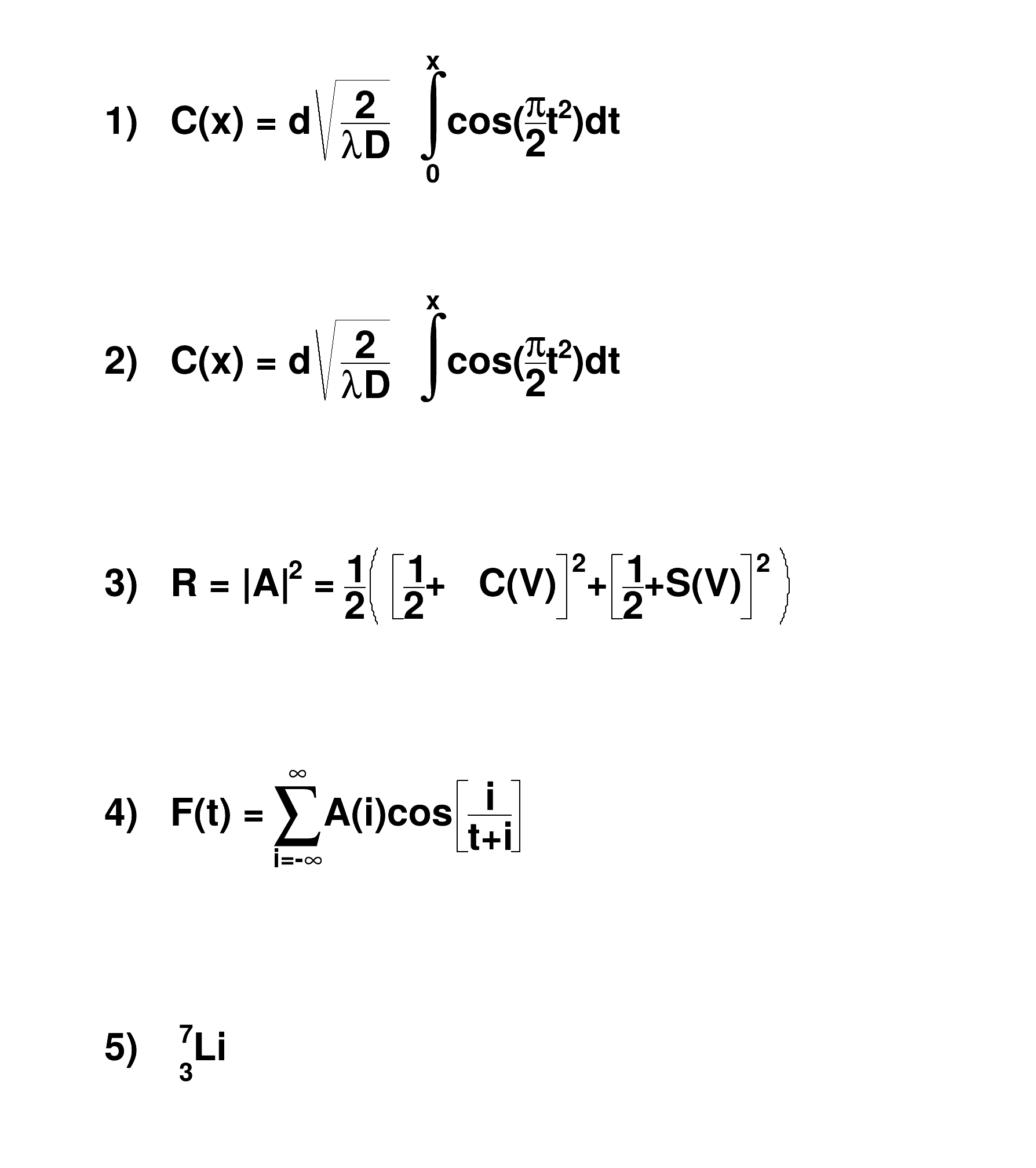ROOT   Reference Guide
Searching...
No Matches
latex.C File Reference

## Detailed DescriptionThis macro draws 5 Latex-style formula in a canvas and prints the canvas as a Postscript file.void latex() {
TCanvas *c1 = new TCanvas("c1","test",600,700);
// write formulas
l.SetTextAlign(12);
l.SetTextSize(0.04);
#int^{x}_{0}cos(#frac{#pi}{2}t^{2})dt");
#int^{x}cos(#frac{#pi}{2}t^{2})dt");
l.DrawLatex(0.1,0.5,"3) R = |A|^{2} = #frac{1}{2}#left(#[]{#frac{1}{2}+\
C(V)}^{2}+#[]{#frac{1}{2}+S(V)}^{2}#right)");
l.DrawLatex(0.1,0.3,
"4) F(t) = #sum_{i=-#infty}^{#infty}A(i)cos#[]{#frac{i}{t+i}}");
l.DrawLatex(0.1,0.1,"5) {}_{3}^{7}Li");
c1->Print("latex.ps");
}
The Canvas class.
Definition TCanvas.h:23
To draw Mathematical Formula.
Definition TLatex.h:18
void Print(Option_t *option="") const override
Dump this line with its attributes.
Definition TLine.cxx:419
return c1
Definition legend1.C:41
TLine l
Definition textangle.C:4

Definition in file latex.C.